* You are viewing the archive for the ‘Pyramid Holons’ Category

## Pyramid Holons

This posting completes the descriptive formula for the Matter-Being Paradigm and, embedded in it, the Holon Theory of Everything based on mapping the Mind—Soul—Spirit Holon onto every branch of knowledge from the Ancient to the Modern. Here the assumption is that all knowledge whether ancient or modern is assumed valid until otherwise disproved.

This posting also completes the descriptions introduced in posting Geons—Holes—Foam –> Matter-Being by examining an application of the Zero Holon

Diagram 1

Holon Pi—Phi—Li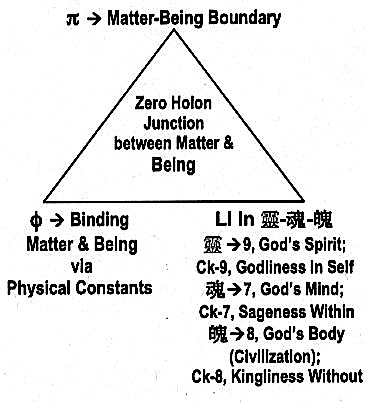Hidden in the dimensions of the Pyramid as shown in Diagrams 4 and 5.

In addition, this posting completes the Ancient [right-brain] and Modern [left-brain] integration formulated in posting Co-Creation Holons; Past-Present, and shown again in the following diagram, namely completing the box refers to as “Atlantian-Egyptian Right-Brain Technology”.

Diagram 2

Integration of Right-Left Brain Sciences and Technology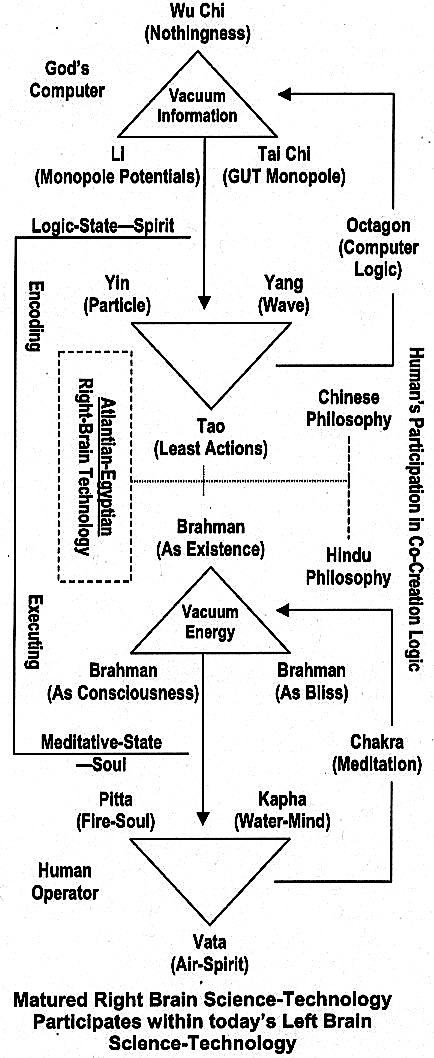The following Diagram 3 completes Diagram 2 for an East-West / Ancient-Modern integration in Right-Left Brain Science and Technology.

Diagram 3

Completing the Integration of Right-Left Brain Sciences and Technology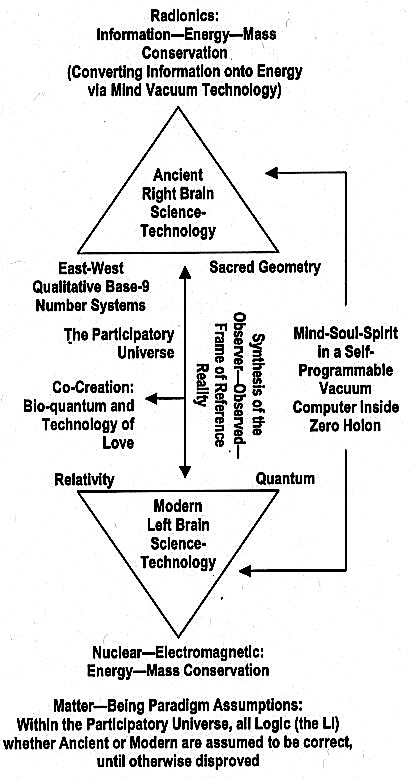Egyptian Science and Technology

Numbers and Measure in Egyptian Sciences

From John Michell’s The Dimensions of Paradise: the Proportions and Symbolic Numbers of Ancient Cosmology:

Ancient science was based like that of today on number, but whereas number is now used in the quantitative sense for secular purposes, the ancients regarded numbers as symbols of the universe, finding parallels between the inherent structure of number and all types of form and motion. Theirs was a very different view of the world from that which now obtains. They inhabited a living universe, a creature of divine fabrication, designed in accordance with reason and thus to some extend comprehensible by the human mind.

From John Michell’s The New View Over Atlantis:

… the Pyramid’s dimensions, as originally intended, can never be defined exactly by measurement alone, for there always remain possible sources of error including faults by the builders and by modern surveyors and the ravages of time. The only means of interpreting the surveyors’ data is though knowledge of the precise values of the builders’ units of measure. Reference to the list of ancient units … prompts the conclusion that the perimeter of the base was designed to measure 3024 ft. [–> 9] making the average lengths of each side equal to 756 ft. {–> 18 –> 9]. The distance round the four sides of the Pyramid is therefore:

3024 ft = 1750 longer Egyptian cubits of 1.728 ft.

= 1760 shorter Egyptian cubits of 1.71818 ft. …

… So the only way of reckoning the height of the finished Pyramid is by measuring the angle of its lope. … about 510 51. …This angle … confirming the claims that the Pyramid monumentalizes the formula of pi, making the height (if 3024 ft. is the perimeter of the base and 22/7 = pi) equal to 280 shorter Egyptian cubits of 481.0909 ft.

The limitations of measurement, however, allow for another interpretation of the relationship between the base and the height. If the angle of slope were very slight less than 51O 51 38” to be exact, it would represent the phi angle rather than pi.

… By this means the functions of both pi and phi could be demonstrated together in [the two diagrams shown below].

Diagram 4

The Yang Pyramid and The Reflective Yin Pyramid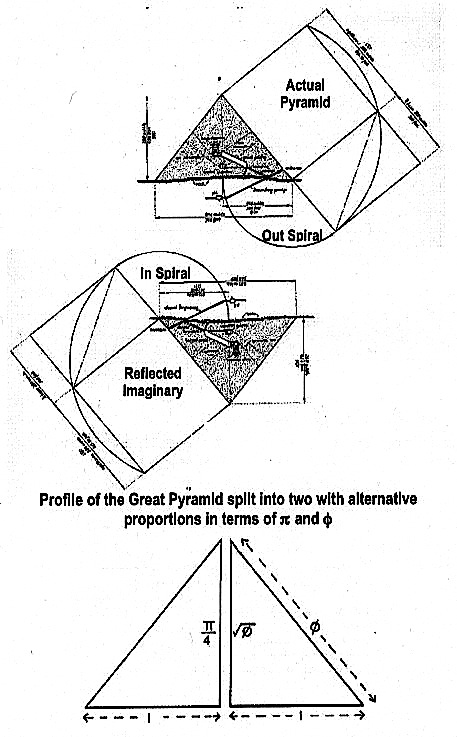and

Diagram 5

Pi—Phi—Li in Pyramid Dimensions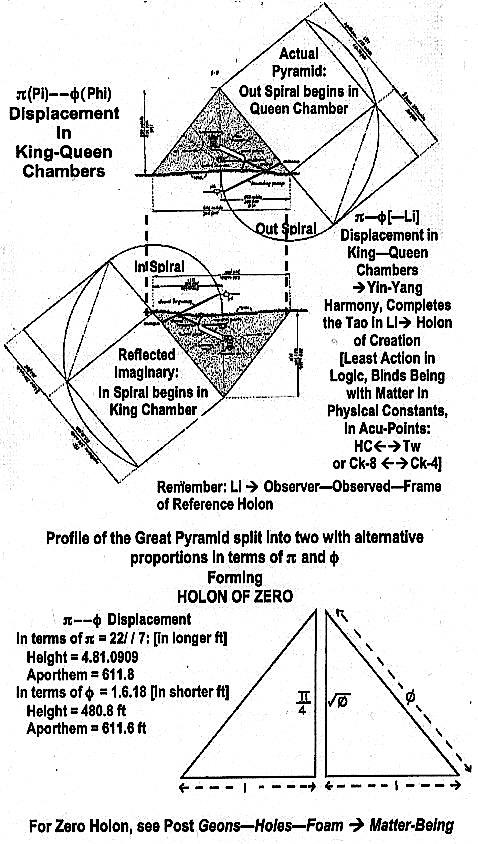The Zero Holon is shown in Diagram 1.

Thus, the power of the Pyramid depends on the Li within the Zero Holon; namely, the Observer—Observed—Frame of Reference Holon.